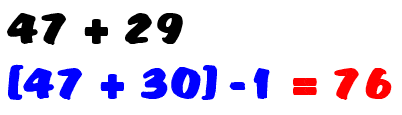# Front End Addition Tricks For Mental Math

Front End Addition Tricks For Mental Math, also known as left-to-right addition, are mental math techniques that allow you to add numbers from left to right rather than the traditional right-to-left method. These tricks can be especially useful for mental calculations, as they break down complex additions into smaller, more manageable steps. Here are some Front End Addition Tricks For Mental Math Left-to-right addition is important to teach BEFORE students learn the traditional algorithm. This is because left-to-right addition focuses on conceptual understanding rather than on the memorization of a series of steps.

## How to Improve at Mental Math : Our Tips And Tricks

Front End Addition Tricks For Mental Math is an excellent skill for children to have, but how, as parents and teachers, do we help them to develop this skill? Luckily, there are many mental math practice strategies and aids to help with this. Take a look below to find out how!

### Splitting into Hundreds, Tens And Units

If your child is confronted with a large addition or subtraction sum, a great strategy to teach them is to break it into smaller parts.

It’s best to break the hundreds, tens, and units up so that they can be added together separately and then Totalled at the end.

### For example, Take a Look at The Following Sum:

541 + 235.

By breaking this down, the numbers can be more easily added together:

500 + 200 = 700, 40 + 30 = 70 and 1 + 5 = 6

These can then be put back together for a final number – 786!

Once your child has gotten the hang of this, they may find it easier to keep the tens and units together and to separate the hundreds, as this makes it easier when the unit adds to over ten. For example, 418 + 513 (400 + 500 = 900, 18 + 13 = 31, meaning that the overall total is 931).

With  front-end-addition  mental math tricks you will be able to work out sums in your head more rapidly – a critical skill in math. Here are examples of some Front End Addition Tricks For Mental Math strategies for addition.

## Break Up the Numbers Strategy Front End Addition Tricks For Mental Math

This strategy is used when regrouping is required.  One of the addends is broken up into its expanded form and added in parts to the other addend.

For example:can be calculated this way: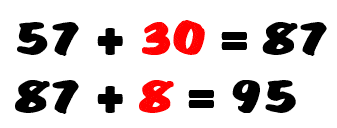## Front-End Strategy

This strategy involves adding the front-end digits  – the hundreds, then the tens and then the ones. For example: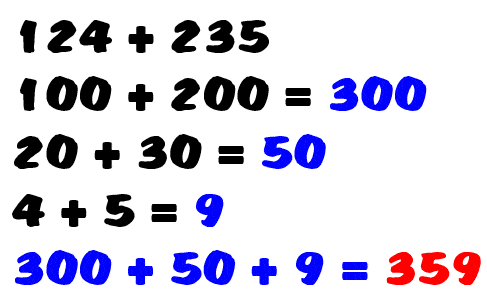## Front-End Estimation

This method involves adding from the left and then grouping the numbers in order to adjust the estimate.  For example: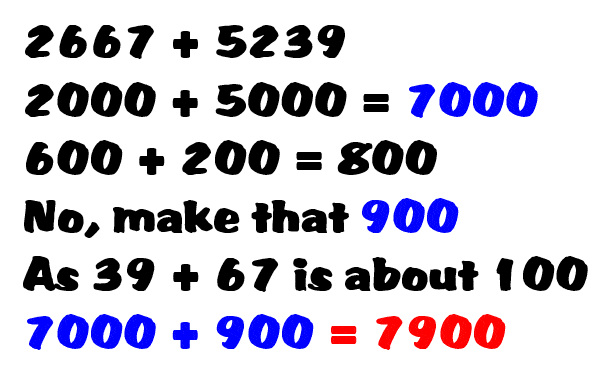## Compatible Number Strategy

Compatible numbers are number pairs that go together to make “friendly” numbers.  That is, numbers that are easy to work with.  For example: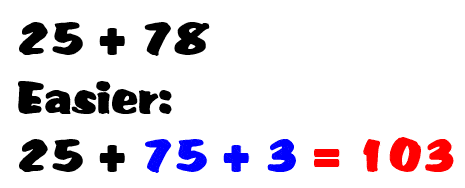## Near Compatible Estimation

Knowledge of the compatible numbers that are used for mental calculations is used for estimation.  For example: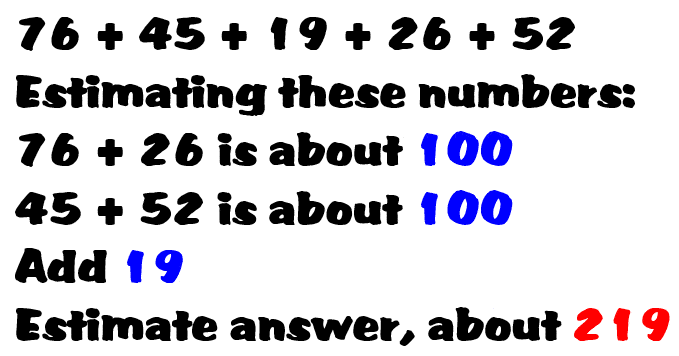## Balancing Strategy

A variation of the compatible number strategy, this method involves taking one or more from one addend and adding it to the other.  For example, 68 + 57 becomes 70 + 55 (add 2 to 68 and take 2 from 57).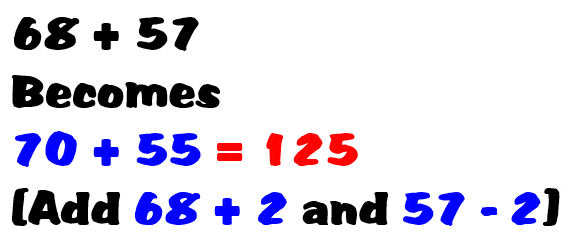## Clustering in Estimation

Clustering involves grouping addends and determining the average.  For example:

when estimating 53 + 47 + 48 + 58 +52, notice that the addends cluster around 50.  The estimate would be 250 (5 x 50)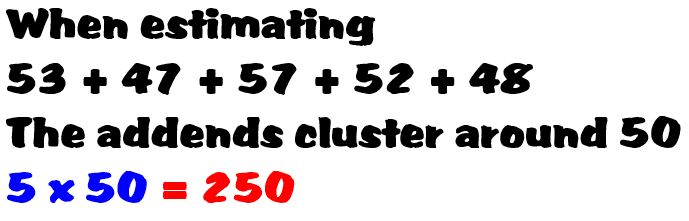## Special Tens Strategy

In the early grades, students learn the number of pairs that total ten – 1 and 9, 2 and 8, 3 and 7, and so on.  These can be extended to such combinations as 10 and 90, 300 and 700, etc.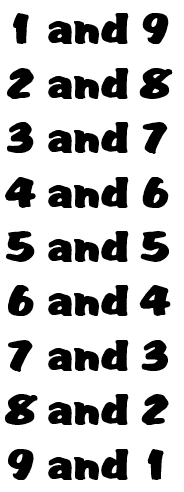## Compensation Strategy

Using the compensation strategy, you substitute a compatible number for one of the numbers so that you can more easily compute mentally.  For example, in doing the calculation 47 + 29 one might think (47 + 30) – 1.